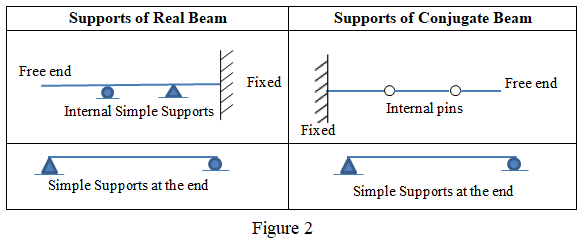# What is Conjugate beam

Conjugate beam is an imaginary beam used to determine the slope and deflection of a beam. The method of conjugate beam was developed by Westergaard in 1921. It was inspired by the works of Otto Mohr and Muller-Breslau related to Moment-Area theorems.

Conjugate beam has the following relationship with the real beam.

1. Length of conjugate beam is equal to the length of real beam.
2. Load on the conjugate beam is the M/EI diagram of the real beam. M/EI diagram is obtained by dividing the bending moment diagram by flexural rigidity (EI) which is the product of modulus of elasticity and moment of inertia.  This loading is downward if the bending moment is negative and upward if the bending moment is positive. See an example in figure 1 given below  for the loading on conjugate beam where the bending moment values are divided by EI from A to B as the flexural rigidity is EI from A to B, whereas bending moment values are divided by 2EI from B to C as flexural rigidity is 2EI from B to C.3. Supports of conjugate beam are related to real beam as (i) simple support at the end of real beam becomes simple support at the end of conjugate beam (ii) fixed support of real beam becomes free end of conjugate beam (iii) free end of real beam becomes fixed support of conjugate beam (iv) simple support at the end of real beam remains as simple supports at the end of conjugate beam (v) intermediate simple support of a real beam becomes internal pin of the conjugate beam, (v) internal pin of real beam becomes simple support of the conjugate beam. An example showing conversion of supports from real beam to conjugate beam is given below in figure 2.4. Shear force of conjugate beam is equal to the slope of real beam at the corresponding point.
5. Bending moment of conjugate beam is equal to the deflection of real beam at the corresponding point.